Next: Completeness in approximation classes Up: Introduction Previous: Terminology for graph problems   Index

### Approximate algorithms and approximation classes

It is well-known that if an NPO problem can be solved in polynomial time, then its corresponding decision problem can also be solved in polynomial time. As a consequence, if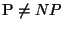, then any NPO problem whose corresponding decision problem is NP-complete is not solvable in polynomial time. In these cases we sacrifice optimality and start looking for approximate solutions computable in polynomial time.

Definition 2

Let A be an NPO problem. Given an instance x and a feasible solution y of x, we define the performance ratio of y with respect to x as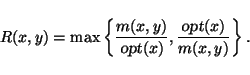The performance ratio is always a number greater than or equal to 1 and is as close to 1 as y is close to the optimum solution.

Definition 3

Let A be an NPO problem and let T be an algorithm that, for any instance x of A, returns a feasible solution T(x) of x. Given an arbitrary function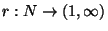, we say that T is an r(n)-approximate algorithm for A if, for any instance x, the performance ratio of the feasible solution T(x) with respect to x verifies the following inequality:If an NPO problem admits an r(n)-approximate polynomial-time algorithm we say that it is approximable within r(n).

Definition 4

An NPO problem A belongs to the class APX if it is approximable within, for some constant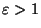.

Definition 5

Let A be an NPO problem. An algorithm T is said to be an approximation scheme for A if, for any instance x of A and for any rational,returns a feasible solution of x whose performance ratio is at most.

Definition 6

An NPO problem A belongs to the class PTAS if it admits a polynomial-time approximation scheme, that is, an approximation scheme whose time complexity is bounded by q(|x|) where q is a polynomial.

Observe that the time complexity of an approximation scheme in the above definition may be of the typeor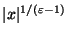where p is a polynomial. Thus, computations withvalues very close to 1 may turn out to be practically unfeasible. This leads us to the following definition.

Definition 7

An NPO problem A belongs to the class FPTAS if it admits a fully polynomial-time approximation scheme, that is, an approximation scheme whose time complexity is bounded bywhere q is a polynomial.

Clearly, the following inclusions hold: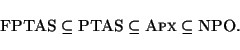It is also easy to see that these inclusions are strict if and only if.Next: Completeness in approximation classes Up: Introduction Previous: Terminology for graph problems   Index
Viggo Kann
2000-03-20